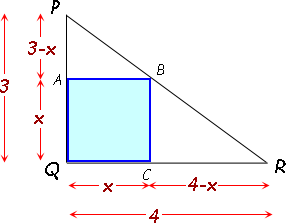SEARCH HOMEMath Central Quandaries & QueriesQuestion from Adrian, a student: Consider a right-angled triangle PQR, where QR is the base and PQ is the height. QR=4cm and PQ=3cm. A square is inscribed in this triangle.Determine the length of one side of the square.Hi Adrian. I started by drawing the diagram, assuming the square shared the vertex Q with the triangle, and then I labelled the other corners of the inscribed square. I called the length of the square x, and used that to determine the other lengths.Let's consider the areas of the shapes. The large triangle PQR is composed of two right triangles PAB and BCR as well as the square. So if you add the areas of these three shapes, you get the area of the large triangle:

Area(PQR) = Area(PAB) + Area(BCR) + Area(AQCB)

Since we know that the area of a triangle is half the base times the height and the area of a square is the side squared, this makes:

(½)(4)(3) = (½)(x)(3 - x) + (½)(4 - x)(x) + x2 .

Solve for x.

Cheers,
Stephen La Rocque.Math Central is supported by the University of Regina and The Pacific Institute for the Mathematical Sciences.